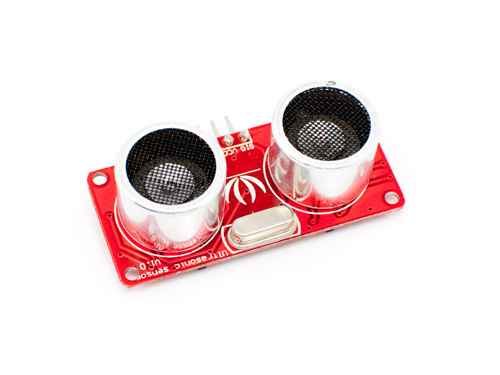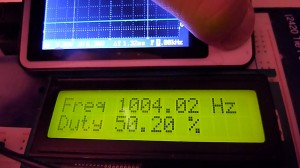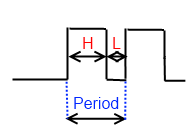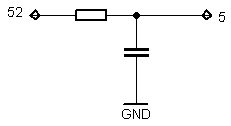# Arduino and ultra sonic range measurement module or how to measure the pulse time with a hardware timer and an interruptSEN136B5B is a Ultra Sonic range measurement from SeedStudio. It can measure the distance by sending a 40k Hz ultra sound impulse via the transmitter  and calculating time needed for echo to reach receiver. Detecting range: 3cm-4m. More information about device.

In the description of module is mentioned that it is compatible with Arduino library, but I decided to write program without using PulseIn command.

# Arduino frequency counter/duty cycle meterThis meter gives the best results at 0 – 1000 Hz  range. It works by measuring square wave total and high period duration using 16 bit hardware counter.As you may know frequency = 1/Period and  Duty Cycle = High period duration/total period duration.

# Very simple Arduino capacitance meter using 16 bit timer and analog comparator

As You could see from video it requires only one external resistor. Meter is very simple, but obviously not very accurate or wide range. Theoretically it could measure in 10 nF – 160 µF range.

How it Works?

Circuit formed from resistor and capacitor (RC circuit) has time constant, it shows time needed to discharge capacitor via resistor to ~37% it’s initial voltage. Calculation of time constant is very simple t=R*C . For 1 k resistor and 47µF capacitor it’s 47ms.My capacitance meter fully charges capacitor, when 16 bit timer is started and capacitor is discharging via resistor (1 k). Capacitor is connected to analog voltage comparator(pin 5), as capacitor voltage drops bellow 1.1 V occurs analog comparator interrupt which triggers timer interrupt.  If resistor is constant, discharge time and capacitance dependency is linear, therefore if You know one rated capacity capacitor discharge time, You could easily calculate another capacitor’s capacitance by measuring time.

Because timer uses only one  /64 clock prescaler measurement range is very narrow. To have wider range there is a always way to make  programmable prescaler, which changes if capacitor value is out of range, or discharge capacitor via range of different resistors.

Also keep in mind that resistor’s resistance depends on environment temperature, consequently and on LCD display showed capacitance. To fix this temperature dependency referenced capacitor should be used. In this case every time both capacitors are charged and discharged separately, but via the same resistor. Capacitance proportion is calculated and if referenced capacitor capacitance is know measured capacitor’s value can be calculated just by division or multiplying.

It’s time for program’s code.

# Arduino 4 digits 7 segments LED countdown timer with buzzer

After sorting out  how works 16 bit hardware timer it is time for 4 digits countdown timer. Having 16 bit timer and  7 segments LED code from earlier only were remaining to write timer’s modes (run/setup) and button’s control code.  After putting  all code to one place there is countdown timer with properties below:

• Maximum 99 minutes 59 seconds countdown interval
• 1 second resolution
• Sound indication with buzzer for finished countdown
• LED indicates running timer, or relay instead  for powering external devices for some period of time
• 2 buttons to set timer and start/pause/reset.

This time without additional code quotation, please find some code explanation within code, so code bellow.

# Examples of using Arduino/Atmega 16 bit hardware timer for digital clock

Arduino Mega with Atmega 1280 has four 16 bit timers, that could be used for various purposes, like time/frequency measurement, control with precise timing, PWM generation. Today I hope to explain how to use timer for clocks, timers (countdown) and other things, where You need µCPU to perform some tasks after precise period of time. I’ll give You two examples:

• Pseudo 1 second timer
• Real 1 second timer

How counter works? It is simple independent  16 bit accumulator, which value increases by 1 at clock cycle. 16 bit means that maximum counter’s value is 65536. When this value is reached counter starts counting from 0 again and gives hardware interrupt. Counter value could be changed any time. This is normal counter mode, Atmega 1280 offers total 14 operating modes.

Pseudo 1 second timer (1.048576 s)